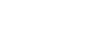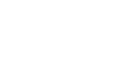# Rayleigh Distribution: Definition, Uses, Mean, Variance

## What is the Rayleigh Distribution?

The Rayleigh distribution is a continuous probability distribution named after the English Lord Rayleigh. It is a special case of the Weibull distribution with a scale parameter of 2. When a Rayleigh is set with a shape parameter (σ) of 1, it is equal to a chi square distribution with 2 degrees of freedom.

The notation X Rayleigh(σ) means that the random variable X has a Rayleigh distribution with shape parameter σ. The probability density function (X > 0) is:Where e is Euler’s number.

As the shape parameter increases, the distribution gets wider and flatter.Rayleigh dist. showing several different shape parameters, σ.

The distribution is widely used:

• In communications theory, to model multiple paths of dense scattered signals reaching a receiver.
• In the physical sciences to model wind speed, wave heights and sound/light radiation.
• In engineering, to measure the lifetime of an object, where the lifetime depends on the object’s age. For example: resistors, transformers, and capacitors in aircraft radar sets.1
• In medical imaging science, to model noise variance in magnetic resonance imaging.

## Variance and Mean (Expected Value) of a Rayleigh Distribution

The expected value (the mean) of a Rayleigh is:How this equation is derived involves solving an integral, using calculus:
The expected value of a probability distribution is:
E(x) = ∫ xf(x)dx.

Solving the integral for you gives the Rayleigh expected value of σ √(π/2)

The variance of a Rayleigh distribution is derived in a similar way, giving the variance formula of:
Var(x) = σ2((4 – π)/2).

## References

:
A 3-Component Mixture: Properties and Estimation in Bayesian Framework. Aslam et. al. Retrieved October 3, 2015. Available here.

CITE THIS AS:
Stephanie Glen. "Rayleigh Distribution: Definition, Uses, Mean, Variance" From StatisticsHowTo.com: Elementary Statistics for the rest of us! https://www.statisticshowto.com/rayleigh-distribution/
---------------------------------------------------------------------------Need help with a homework or test question? With Chegg Study, you can get step-by-step solutions to your questions from an expert in the field. Your first 30 minutes with a Chegg tutor is free!